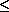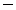### Ticker

6/recent/ticker-posts

# Production in the Short-run & Long-run

Production Functions
The production function relates the quantity of factor inputs used by a business to the amount of output that result. We use three measures of production and productivity.
• Total product (or total output). In manufacturing industries such as motor vehicles and DVD players, it is straightforward to measure how much output is being produced. But in service or knowledge industries, where output is less “tangible” it is harder to measure productivity.
• Average product measures output per-worker-employed or output-per-unit of capital.
• Marginal product is the change in output from increasing the number of workers used by one person, or by adding one more machine to the production process in the short run.

The length of time required for the long run varies from sector to sector. In the nuclear power industry for example, it can take many years to commission new nuclear power plant and capacity. This is something the UK government has to consider as it reviews our future sources of energy.
Short Run Production Function
• The short run is a time period where at least one factor of production is in fixed supply. A business has chosen it’s scale of production and must stick with this in the short run
• We assume that the quantity of plant and machinery is fixed and that production can be altered by changing variable inputs such as labour, raw materials and energy.
• The time periods used differ from one industry to another; for example, the short-run in the electricity generation industry differs from local sandwich bars. If you are starting out in business with a new venture selling sandwiches and coffees to office workers, how long is your long run? It could be as short as a few days – enough time to lease a new van and a sandwich-making machine!
Diminishing Returns
• In the short run, the law of diminishing returns states that as we add more units of a variable input to fixed amounts of land and capital, the change in total output will at first rise and then fall.
• Diminishing returns to labour occurs when marginal product of labour starts to fall. This means that total output will be increasing at a decreasing rate.
What might cause marginal product to fall? One explanation is that, beyond a certain point, new workers will not have as much capital equipment to work with so it becomes diluted among a larger workforce.
In the following numerical example, we assume that there is a fixed supply of capital (20 units) to which extra units of labour are added.
• Initially, marginal product is rising – e.g. the 4th worker adds 26 to output and the 5th worker adds 28 and the 6th worker increases output by 29.
• Marginal product then starts to fall. The 7th worker supplies 26 units and the 8th worker just 20 added units. At this point production demonstrates diminishing returns.
• Total output will continue to rise as long as marginal product is positive
• Average product will rise if marginal product > average product
 The Law of Diminishing Returns Capital Input Labour Input Total Output Marginal Product Average Product of Labour 20 1 5 5 20 2 16 11 8 20 3 30 14 10 20 4 56 26 14 20 5 85 28 17 20 6 114 29 19 20 7 140 26 20 20 8 160 20 20 20 9 171 11 19 20 10 180 9 18Average product rises as long as marginal product is greater than the average – e.g. when the seventh worker is added the marginal gain in output is 26 and this drags the average up from 19 to 20 units. Once marginal product is below the average as it is with the ninth worker employed then the average must decline.
Criticisms of the Law of Diminishing Returns
• How realistic is this assumption of diminishing returns? Surely ambitious and successful businesses will do their level best to avoid such a problem emerging?
• It is now widely recognised that the effects of globalisation and the ability of trans-national businesses to source their inputs from more than one country and engage in transfers of business technology, makes diminishing returns less relevant as a concept.
• Many businesses are multi-plant meaning that they operate factories in different locations – they can switch output to meet changing demand.
Long Run Production - Returns to Scale
In the long run, all factors of production are variable. How the output of a business responds to a change in factor inputs is called returns to scale.
 Numerical example of long run returns to scale Units of Capital Units of Labour Total Output % Change in Inputs % Change in Output Returns to Scale 20 150 3000 40 300 7500 100 150 Increasing 60 450 12000 50 60 Increasing 80 600 16000 33 33 Constant 100 750 18000 25 13 Decreasing
• When we double the factor inputs from (150L + 20K) to (300L + 40K) then the percentage change in output is 150% - there are increasing returns to scale.
• When the scale of production is changed from (600L + 80K0 to (750L + 100K) then the percentage change in output (13%) is less than the change in inputs (25%) implying a situation of decreasing returns to scale.
• Increasing returns to scale occur when the % change in output > % change in inputs
• Decreasing returns to scale occur when the % change in output < % change in inputs
• Constant returns to scale occur when the % change in output = % change in inputs
The nature of the returns to scale affects the shape of a business’s long run average cost curve.

Finding an optimal mix between labour and capital
In the long run businesses will be looking to find an output that combines labour and capital in a way that maximises productivity and therefore reduces unit costs towards their lowest level. This may involve a process of capital-labour substitution where capital machinery and new technology replaces some of the labour input.
In many industries over the years we have seen a rise in the capital intensity of production - good examples include farming, banking and retailing.
Robotic technology is extensively used in many manufacturing / assembly industries such as cars and semi-conductors. The image above is of a Ford car assembly factory in India.

## Example of long run and short run cost functions

### Example: a production function with fixed proportions

Consider the fixed proportions production function F (z1z2) = min{z1z2} (one worker and one machine produce one unit of output). The long run total cost function for this production function is given by
TC(y,w1,w2) = w1y + w2y = (w1 + w2)y.
Its short run total cost of production when the amount of input 2 is fixed at k is
 STCk(y) = w1y + w2k if ykif y > k.
These functions are shown in the following figure.### Example: a production function in which the inputs are perfect substitutes

Consider the production function F (z1, z2) = z1 + z2, in which the inputs are perfect substitutes. The long run total cost function for this production function is given by
 w1y if w1 < w2 TC(y,w1,w2) = wy if w1 = w2 = w w2y if w1 > w2
Its short run total cost of production when the amount of input 2 is fixed at k is
 STCk(y) = w2k if yk w1(yk) + w2k if y > k.
Note that in this case if w1 < w2 then there is no level of output for which short run total cost is equal to the long run total cost. In this case the firm would like to use only input 1 to produce any output, so if k > 0 there is no output for which the short run total cost is equal to the long run total cost. The short and long run cost functions in this case are shown in the following figure.### Example: a Cobb-Douglas production function

Consider the production function F (z1z2) = z11/2z21/2. The long run total cost function for this production function is given by
TC(y,w1,w2) = 2y(w1w2)1/2.
Its short run total cost of production when the amount of input 2 is fixed at k is
STCk(y) =w1y2/k + w2k.
Note that TC is a linear function of y while STC is a quadratic function. (Remember that w1 and w2 are fixed.) The functions are shown in the following figure.